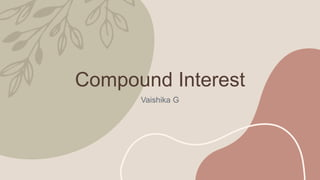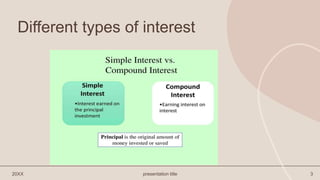Ce diaporama a bien été signalé.
Le téléchargement de votre SlideShare est en cours. ×

# Compound Interest.pptx

Publicité
Publicité
Publicité
Publicité
Publicité
Publicité
Publicité
Publicité
Publicité
Publicité
PublicitéChargement dans…3
×

## Consultez-les par la suite

1 sur 11 Publicité

# Compound Interest.pptx

Compound interest is the interest imposed on a loan or deposit amount. It is the most commonly used concept in our daily existence. The compound interest for an amount depends on both Principal and interest gained over periods. This is the main difference between compound and simple interest.

Suppose we observe our bank statements, we generally notice that some interest is credited to our account every year. This interest varies with each year for the same principal amount. We can see that interest increases for successive years. Hence, we can conclude that the interest charged by the bank is not simple interest; this interest is known as compound interest or CI. In this article, you will learn what is compound interest, the formula and the derivation to calculate compound interest when compounded annually, half-yearly, quarterly, etc. Also, one can understand why the return on compound interest is more than the return on simple interest through the examples given based on real-life applications of compound interest here.

Compound interest is the interest imposed on a loan or deposit amount. It is the most commonly used concept in our daily existence. The compound interest for an amount depends on both Principal and interest gained over periods. This is the main difference between compound and simple interest.

Suppose we observe our bank statements, we generally notice that some interest is credited to our account every year. This interest varies with each year for the same principal amount. We can see that interest increases for successive years. Hence, we can conclude that the interest charged by the bank is not simple interest; this interest is known as compound interest or CI. In this article, you will learn what is compound interest, the formula and the derivation to calculate compound interest when compounded annually, half-yearly, quarterly, etc. Also, one can understand why the return on compound interest is more than the return on simple interest through the examples given based on real-life applications of compound interest here.

Publicité
Publicité

### Compound Interest.pptx

1. 1. Compound Interest Vaishika G
2. 2. What is interest? 20XX presentation title 2 Interest refers to the cost of borrowing money or the reward for lending money. Typically, banks charge interest on money borrowed on top of the expected repayment of the principal. At the same time, banks also pay interest on depositors’ funds in savings and investment accounts. They do so to entice more deposits, which they use for on-lending to customers, charging a higher interest rate than they pay depositors.
3. 3. Different types of interest 20XX presentation title 3
4. 4. Amount of interest over the years 20XX presentation title 4
5. 5. 20XX presentation title 5 Compound Interest Compound interest is the interest calculated on the principal and the interest accumulated over the previous period. It is different from simple interest, where interest is not added to the principal while calculating the interest during the next period. In Mathematics, compound interest is usually denoted by C.I.
6. 6. • Where, • A = amount • P = principal • r = rate of interest • n = number of times interest is compounded per year • t = time (in years) 20XX presentation title 6 Formula
7. 7. Formula This formula is also called periodic compounding formula. Here, • A represents the new principal sum or the total amount of money after compounding period • P represents the original amount or initial amount • r is the annual interest rate • n represents the compounding frequency or the number of times interest is compounded in a year • t represents the number of years
8. 8. Derivation 20XX presentation title 8
9. 9. presentation title 9
10. 10. 10
11. 11. thank you Vaishika G